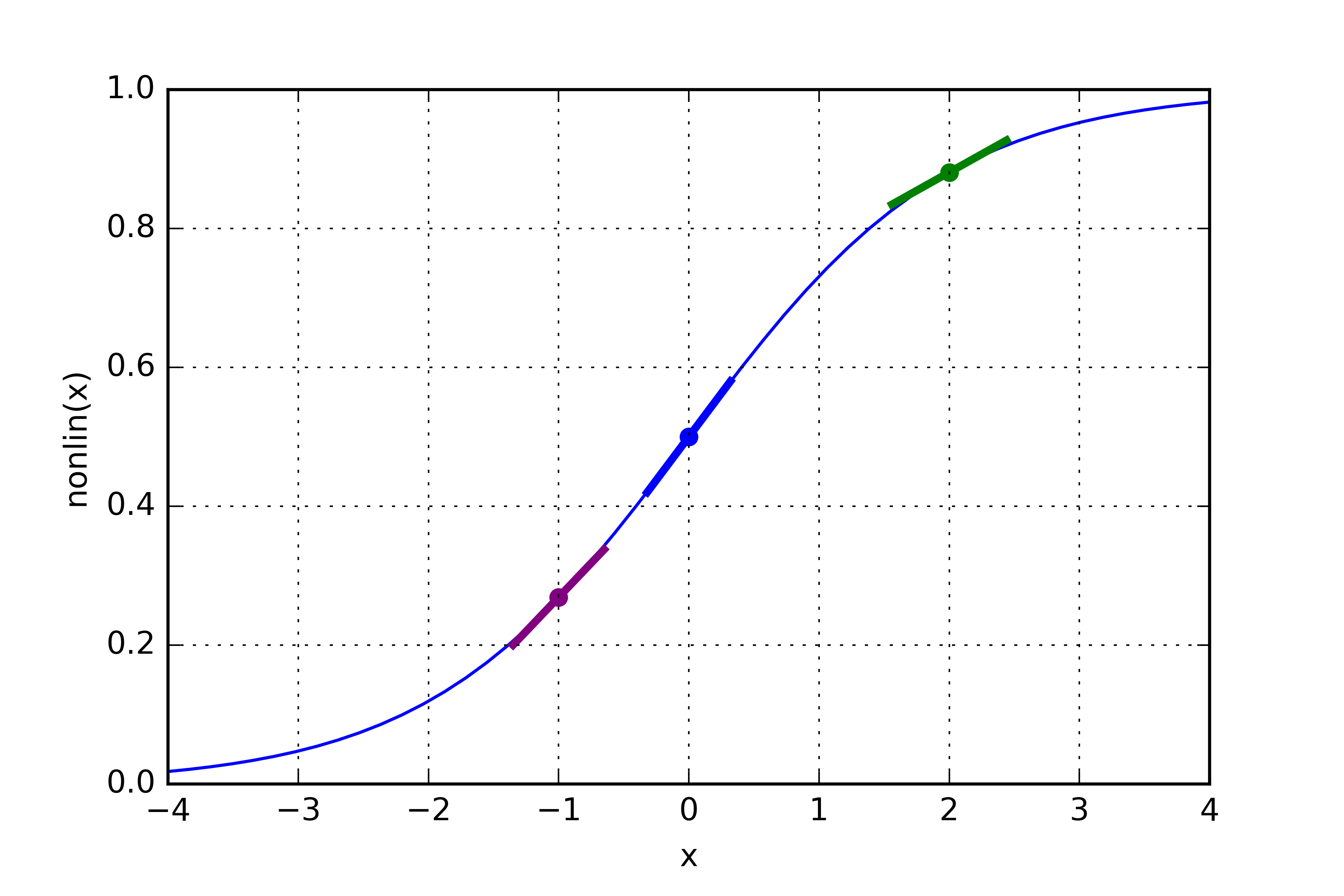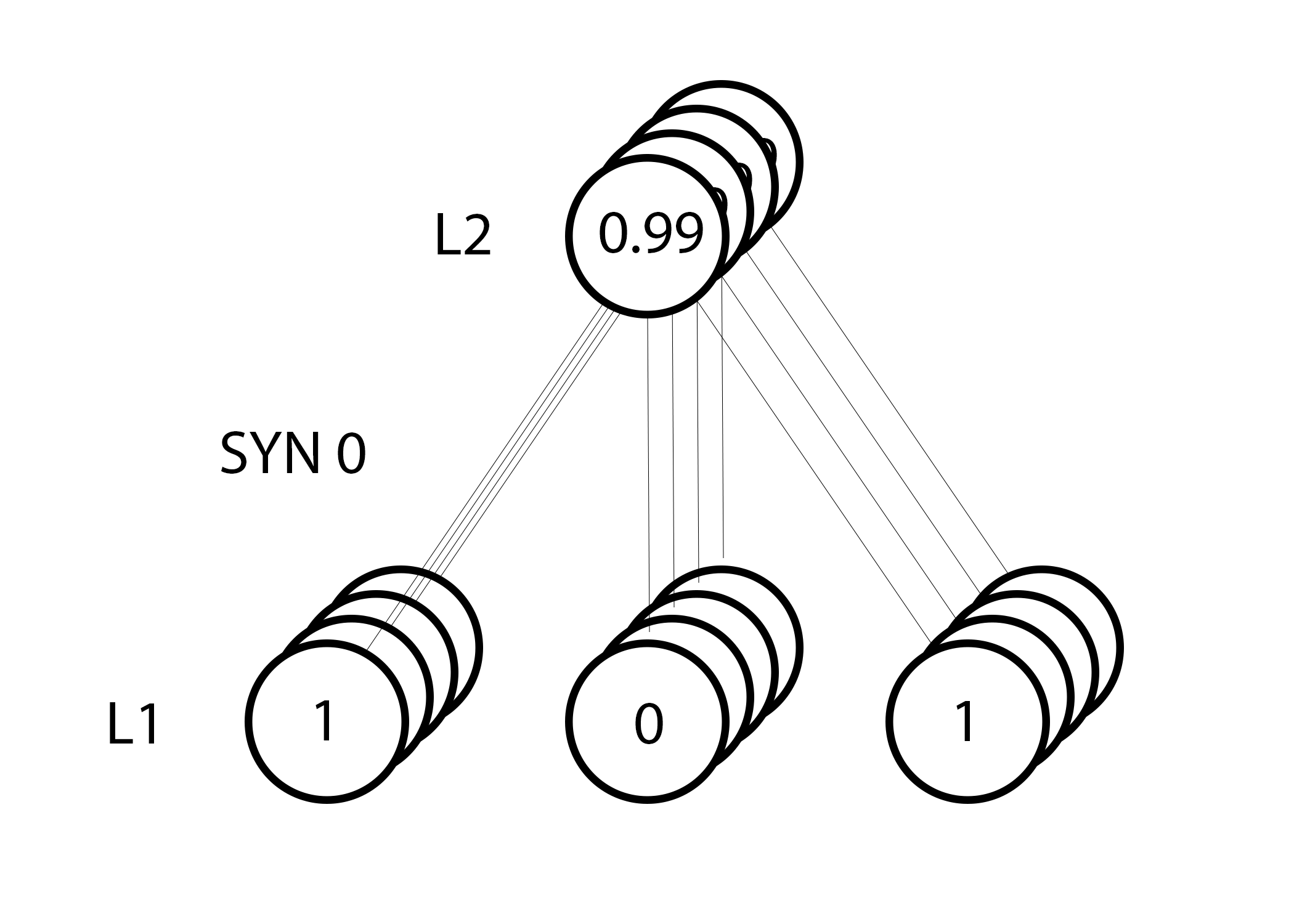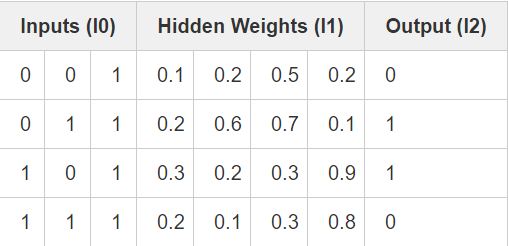BP（Back Propagation）神经网络是1986年由Rumelhart和McCelland为首的科学家小组提出，是一种按误差逆传播算法训练的多层前馈网络，是目前应用最广泛的神经网络模型之一。BP网络能学习和存贮大量的输入-输出模式映射关系，而无需事前揭示描述这种映射关系的数学方程。它的学习规则是使用梯度下降法，通过反向传播来不断调整网络的权值和阈值，使网络的误差平方和最小。BP神经网络模型拓扑结构包括输入层（input）、隐层(hidden layer)和输出层(output layer)。

X = np.array([ [0,0,1],[0,1,1],[1,0,1],[1,1,1] ])
y = np.array([[0,1,1,0]]).T
syn0 = 2*np.random.random((3,4)) - 1
syn1 = 2*np.random.random((4,1)) - 1
for j in xrange(60000):
l1 = 1/(1+np.exp(-(np.dot(X,syn0))))
l2 = 1/(1+np.exp(-(np.dot(l1,syn1))))
l2_delta = (y - l2)*(l2*(1-l2))
l1_delta = l2_delta.dot(syn1.T) * (l1 * (1-l1))
syn1 += l1.T.dot(l2_delta)
syn0 += X.T.dot(l1_delta)


11行代码就实现了一个神经网络....然而，这也是一个过于简洁的神经网络。下面我就将分步讲解这个神经网络的实现，以帮助读者了解并使用BP反向传播算法。

# 第一部分: 简洁的神经网络

InputsOutput
0010
1111
1011
0110

## 两层的神经网络

import numpy as np

# sigmoid function
def nonlin(x,deriv=False):
if(deriv==True):
return x*(1-x)
return 1/(1+np.exp(-x))

# input dataset
X = np.array([  [0,0,1],
[0,1,1],
[1,0,1],
[1,1,1] ])

# output dataset
y = np.array([[0,0,1,1]]).T

# seed random numbers to make calculation
# deterministic (just a good practice)
np.random.seed(1)

# initialize weights randomly with mean 0
syn0 = 2*np.random.random((3,1)) - 1

for iter in xrange(10000):

# forward propagation
l0 = X
l1 = nonlin(np.dot(l0,syn0))

# how much did we miss?
l1_error = y - l1

# multiply how much we missed by the
# slope of the sigmoid at the values in l1
l1_delta = l1_error * nonlin(l1,True)

# update weights
syn0 += np.dot(l0.T,l1_delta)

print "Output After Training:"
print l1


Output After Training:

[[ 0.00966449]

[ 0.00786506]

[ 0.99358898]

[ 0.99211957]]


X输入数据集，形式为矩阵，每 1 行代表 1 个训练样本。
y输出数据集，形式为矩阵，每 1 行代表 1 个训练样本。
l0网络第 1 层，即网络输入层。
l1网络第 2 层，常称作隐藏层。
syn0第一层权值，突触 0 ，连接 l0 层与 l1 层。
*元素相乘，故两等长向量相乘等同于其对等元素分别相乘，结果为同等长度的向量。
-元素相减，故两等长向量相减等同于其对等元素分别相减，结果为同等长度的向量。
x.dot(y)如果x 和 y 为向量，则进行点积操作；如果均为矩阵，则进行矩阵相乘操作；若其中之一为矩阵，则进行向量与矩阵相乘操作。

• 对比l1层在首次迭代和最后一次迭代的状态
• 观察"nonlin" 函数， 正是它将一个概率值作为输出提供给了我们
• 观察l1_error在迭代中是如何变化的
• 将第36行拆开，这里有大部分的秘密武器
• 观察第39行，网络中所有的操作都是在为这步运算作准备(4 x 3) dot (3 x 1) = (4 x 1)


### 求导

nonlin(l1,True)### 整体认知：误差项加权导数值

l1_delta = l1_error * nonlin(l1,True)


“误差项加权导数值”这个名词在数学上还有更为严谨的描述，但是我觉得这个定义准确地捕捉到了算法的意图。 l1_error（4,1）大小的矩阵，nonlin（l1,True）返回的便是（4,1）的矩阵。而我们所做的就是将其“逐元素地”相乘，得到的是一个（4,1）大小的矩阵 l1_delta ，它的每一个元素就是元素相乘的结果。InputsOutput
0010
1111
1011
0110

# 第二部分：一个稍显复杂的问题

InputsOutput
0010
1111
1011
0110## 我们的策略## 3层神经网络

import numpy as np

def nonlin(x,deriv=False):
if(deriv==True):
return x*(1-x)

return 1/(1+np.exp(-x))

X = np.array([[0,0,1],
[0,1,1],
[1,0,1],
[1,1,1]])

y = np.array([,
,
,
])

np.random.seed(1)

# randomly initialize our weights with mean 0
syn0 = 2*np.random.random((3,4)) - 1
syn1 = 2*np.random.random((4,1)) - 1

for j in xrange(60000):

# Feed forward through layers 0, 1, and 2
l0 = X
l1 = nonlin(np.dot(l0,syn0))
l2 = nonlin(np.dot(l1,syn1))

# how much did we miss the target value?
l2_error = y - l2

if (j% 10000) == 0:
print "Error:" + str(np.mean(np.abs(l2_error)))

# in what direction is the target value?
# were we really sure? if so, don't change too much.
l2_delta = l2_error*nonlin(l2,deriv=True)

# how much did each l1 value contribute to the l2 error (according to the weights)?
l1_error = l2_delta.dot(syn1.T)

# in what direction is the target l1?
# were we really sure? if so, don't change too much.
l1_delta = l1_error * nonlin(l1,deriv=True)

syn1 += l1.T.dot(l2_delta)
syn0 += l0.T.dot(l1_delta)